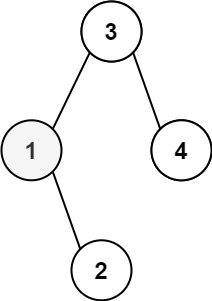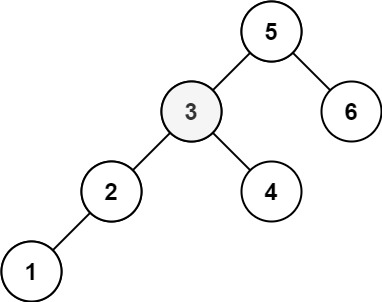# 230. Kth Smallest Element in a BST

Medium
Given the `root` of a binary search tree, and an integer `k`, return the `kth` smallest value (1-indexed) of all the values of the nodes in the tree.
Example 1:Input: root = [3,1,4,null,2], k = 1
Output: 1
Example 2:Input: root = [5,3,6,2,4,null,null,1], k = 3
Output: 3
Constraints:
• The number of nodes in the tree is `n`.
• `1 <= k <= n <= 104`
• `0 <= Node.val <= 104`
Follow up: If the BST is modified often (i.e., we can do insert and delete operations) and you need to find the kth smallest frequently, how would you optimize?

### 解題

/**
* Definition for a binary tree node.
* type TreeNode struct {
* Val int
* Left *TreeNode
* Right *TreeNode
* }
*/
func kthSmallest(root *TreeNode, k int) int {
ans := 0
var helper func(*TreeNode)
helper = func(root *TreeNode){
if root == nil {
return
}
helper(root.Left)
k--
if k == 0 {
ans = root.Val
}
helper(root.Right)
}
helper(root)
return ans
}